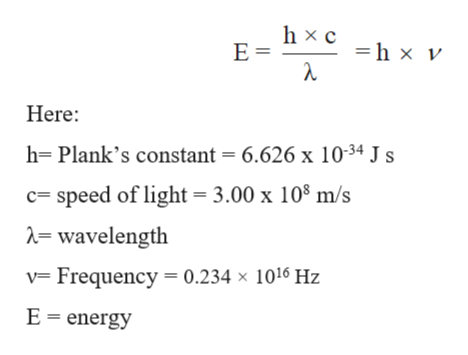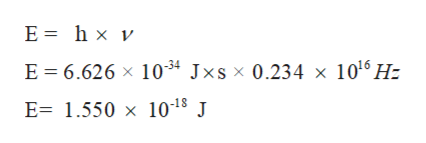# A light photon with a frequency of at least 0.234 × 1016 Hz is required to split apart dinitrogen according to the equation:N2(g) → 2N(g)Calculate the energy (kJ) required to break apart a mole of dinitrogen. Express answer in scientific notation.

Question
15 views
 A light photon with a frequency of at least 0.234 × 1016 Hz is required to split apart dinitrogen according to the equation: N2(g) → 2N(g) Calculate the energy (kJ) required to break apart a mole of dinitrogen. Express answer in scientific notation.
check_circle

Step 1

Electromagnetic spectrum involves different radiations from the lowest to the highest frequency values. Some common radiations which are part of electromagnetic radiations are infrared radiation, visible light, ultraviolet radiation, X-rays, and gamma rays.

Step 2

The relation between energy and wavelength is:help_outlineImage Transcriptionclosehx c Е- h x v Here h- Plank's constant = 6.626 x 1034 Js c= speed of light 3.00 x 108 m/s = wavelength v Frequency 0.234 x 1016 Hz E energy fullscreen
Step 3

Substitute all values to calculate energy:

h= Plank’s constant = 6.626 x 10-34...help_outlineImage TranscriptioncloseE= hx v E 6.626 x 1034 Jxsx 0.234 x 1016 H: E 1.550 x 1018 J fullscreen

### Want to see the full answer?

See Solution

#### Want to see this answer and more?

Solutions are written by subject experts who are available 24/7. Questions are typically answered within 1 hour.*

See Solution
*Response times may vary by subject and question.
Tagged in

### Chemical Thermodynamics